## All the COUNT Functions in Excel

When it comes to reporting and analysis, the COUNT functions in Excel are a necessity. Very often we need to export reports from a CRM. It could be a report on clients, on open tasks or service activities like below and we need to do analysis and create summary reports with it: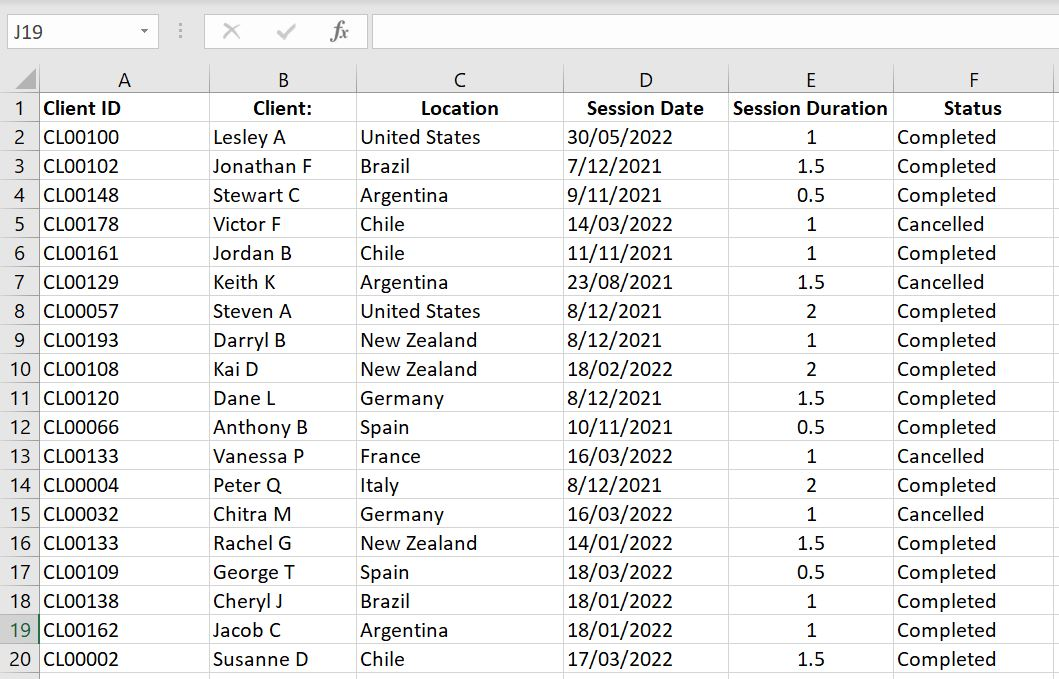In this article we will go through the COUNTIF and COUNTIFS, COUNT, COUNTA and COUNTBLANK functions.

## COUNTIF and COUNTIFS

Do you often export reports from a CRM? And the raw data reports have a full list of entries like the image above. What is the easiest way to calculate how many sessions a particular client attended? How many sessions did a particular client cancelled? How many sessions did a client attend within a specified time period? COUNTIF and COUNTIFS are the perfect functions in Excel.

• =COUNTIF(range, criteria)
• This function counts the number of times a particular value appears in a range of cells. In this function, range is the range of cells we want Excel to search in and criteria is the value Excel is looking for in that range
• =COUNTIFS(criteria_range1, criteria1, [criteria_range2, criteria2], [criteria_range3, criteria3]…)
• This function is the same as COUNTIF except we can set more than one condition. In this function, we first enter the range of cells we want Excel to search in and then set the value Excel needs to look for. And we can keep going by setting another range of cells and another criteria and so on…
• Note that criteria_range2, criteria2, criteria_range3 and criteria3 are in square brackets in the function “[ ]” – that is because they are optional. Technically we can set one criteria_range and one criteria, in which case COUNTIFS and COUNTIF are exactly the same.

In the example below, we have =COUNTIF(\$B:\$B, H3) which is to have Excel search through Column B for the value in Cell H3 (“Jonathan F”).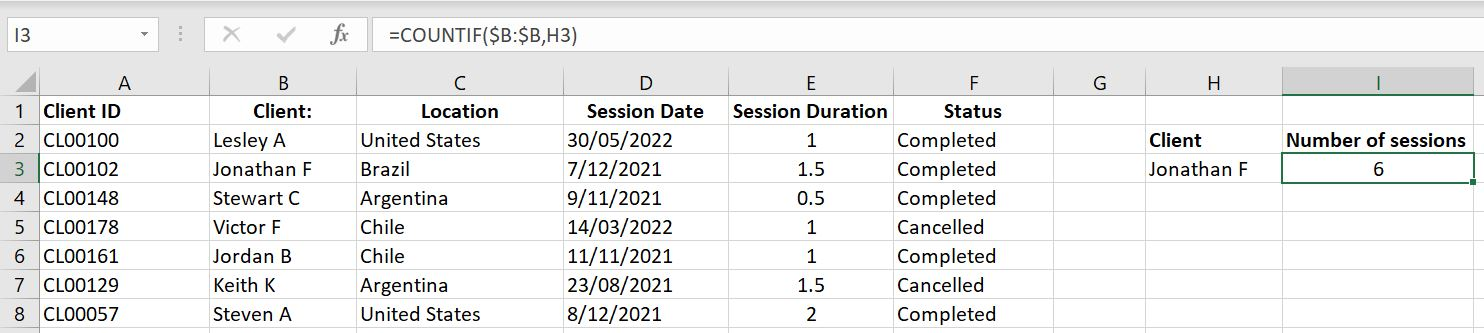=COUNTIF(\$B:\$B, H3)

Using the COUNTIF Function is simple and very useful. But there is one tip to keep in mind:

COUNTIFS is not much different to COUNTIF. Let’s have a look at the example below: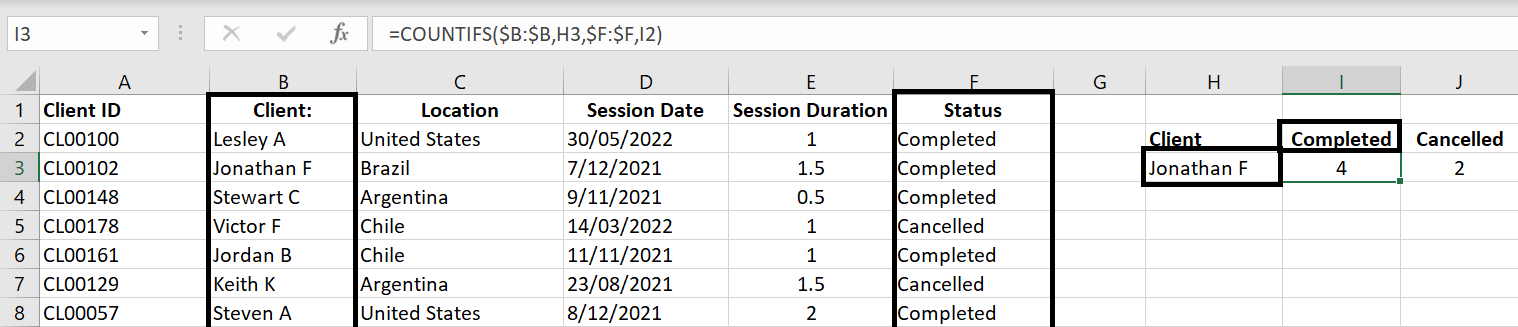=COUNTIFS(\$B:\$B, H3, \$F:\$F, I2)

Here we entered multiple conditions. We want Excel to search through Column B to find the client’s name and also search through Column F to only count the “Completed” status. And of course we can add even more conditions to it. For example, how many sessions did Jonathan cancel that is of a one-hour duration?

### COUNTIF and COUNTIFS with >, >=, < or <=

So far we’ve only looked at COUNTIF and COUNTIFS with conditions where the criteria matches exactly with the range of cells specified. But what if we want to count the number of cells where value is greater or less than a certain number? For example what if we want to count the number of sessions that is longer than one hour?

To demonstrate this, we’ve added clients’ ages in the data and here we want to find out the age demographics of our clients by setting age brackets such as “0 to 18”, “19 to 24”, “25 to 34” and so on: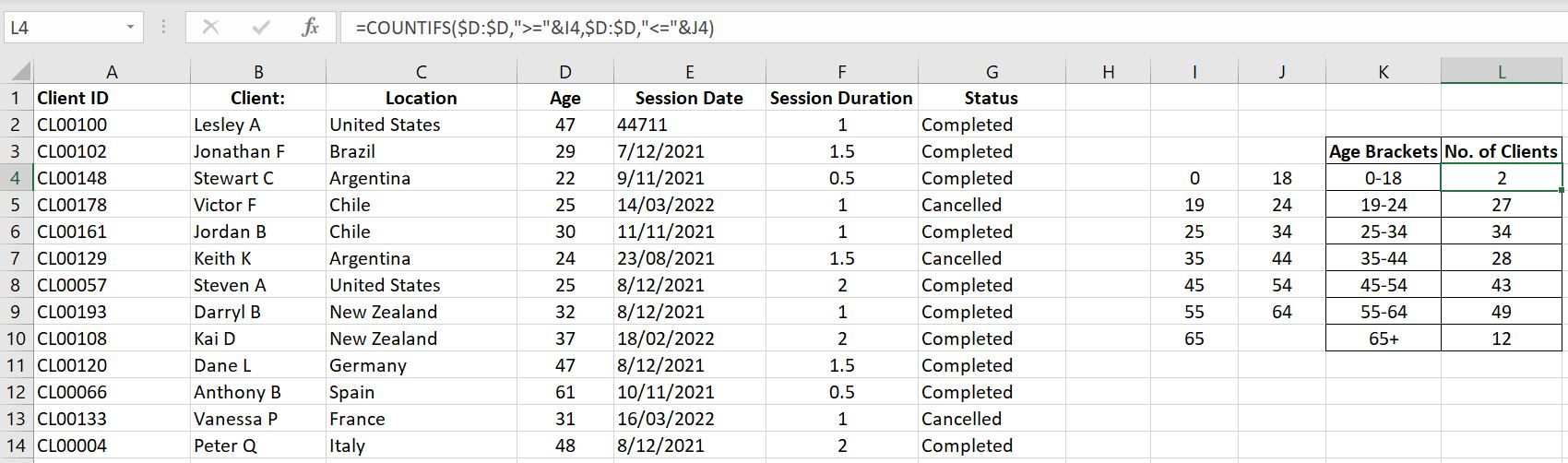Let’s break this down. For age bracket 0 to 18, the formula is:

• =COUNTIFS(\$D:\$D, “>=”&I4, \$D:\$D, “<=”&J4)

First condition is to look through Column D (\$D:\$D) for any value greater or equal to (“>=”&) 0 (Cell I4). And the second condition is to then look through Column D (\$D:\$D) again but this time for any value less than or equal to (“<=”&) 18 (Cell J4). The result is that we are counting the number of cells in Column D which are between 0 to 18 inclusive.

### COUNT functions and SUM

What’s fun with Excel now is that we can now enter an array as the criteria. What that means is Excel will count each criteria in the array for us and list the results one by one. It means you only need to enter the formula once and do not need to autofill or copy the formula down. For example: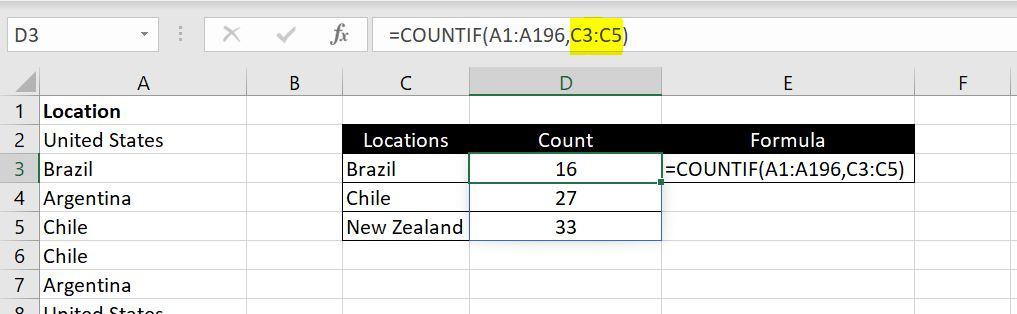=COUNTIF(A1:A196, C3:C5)

Notice in the example above, we entered “C3:C5” as the criteria. And what that does is Excel would calculate the COUNTIF function for C3, C4 and C5 and list the results down automatically in D3:D5.

And to make things even simpler, instead of doing the COUNTIF function multiple times and then calculating the total at the end, we can now wrap a SUM function around a COUNTIF function. This will help if you don’t care what the COUNTIF result is for each criteria and only want to know the total: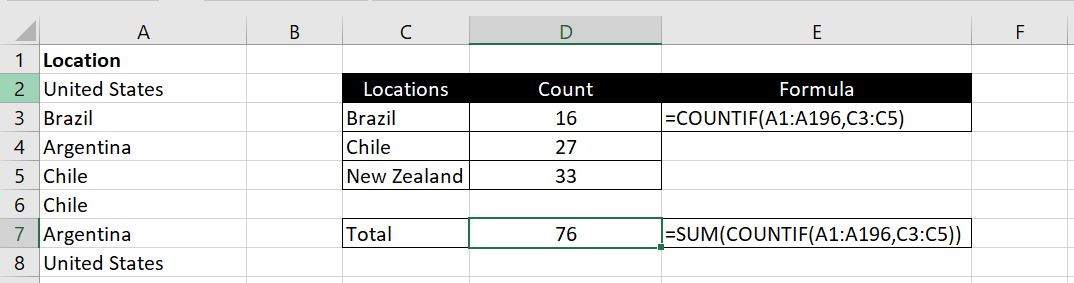=SUM(COUNTIF(A1:A196, C3:C5)

In the example above, we did not need to calculate the COUNTIF function individually for “Brazil”, “Chile” and “New Zealand”. In D7, we can calculate it in one formula by wrapping the SUM function around the COUNTIF function.

## COUNTBLANK

COUNTBLANK as the name suggests counts the number of empty cells in a range specified. This is useful in checking the data to see if there are any blanks. And will give us a chance to fix these data gaps in our CRM.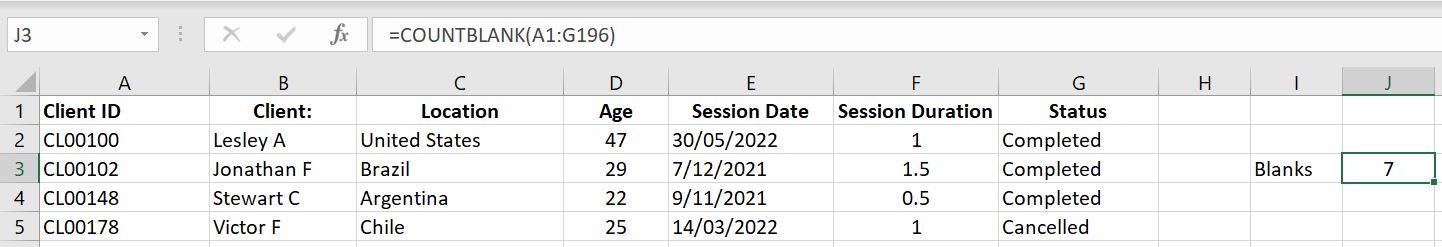The function is incredibly simple to use:

• =COUNTBLANK(the range of cells you want to check if they are empty)

Once again we will just need to be careful. A cell with a space in it ” ” is not blank according to Excel.

## COUNTA and COUNT

• COUNTA: counts the number of cells in the specified range that are not blank
• COUNT: counts the number of cells that contain numbers (0 is a number. Negative numbers are also numbers. Decimals are also numbers)

Consider this set of random data in range A1:A8: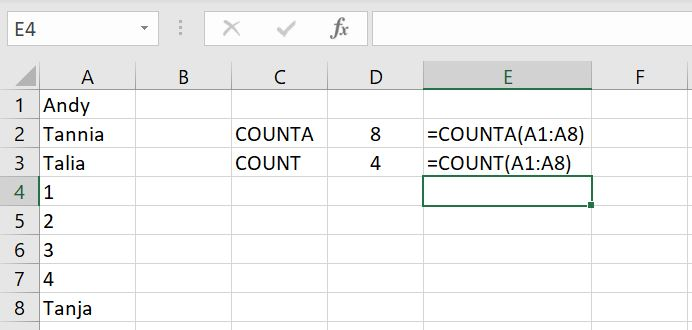=COUNTA(A1:A8)

=COUNT(A1:A8)

COUNTA returns 8 because all 8 cells have data in them. COUNT returns 4 because only 4 of them are numbers.

This little example below show that COUNTA function will also count any cells as not empty if it has formulas in them. In this case, “” is technically equivalent to a blank but COUNTA will still count it as containing data.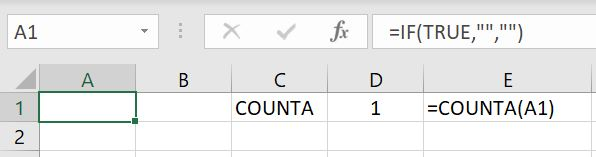You would think that using COUNTA and COUNTBLANK, you would always get the total number of entries. But the example above shows that it doesn’t. COUNTA will count A1 above as 1 (because it has a formula in it although the formula returns a “” result) and COUNTBLANK will also count A1 as 1: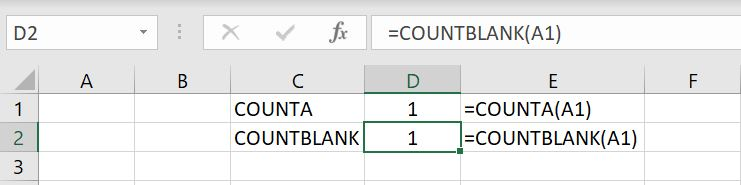Cell A1: =IF(TRUE, “”, “”)

With one cell (A1), both functions are returning 1 as a result. Adding them up, you would get a total of 2 although there’s only one cell. So we just need to keep in mind this is how COUNTA function works. As long as there is some value or formula entered into the cell, COUNTA will count it as a non-empty cell. It makes sense. The irony here is that the formula we put in is returning an empty cell.

We hope you now understand how and when to use each COUNT functions. If there’s more you would like to know about or if there’s more you feel we should add on this topic, please leave a comment below!